Selina Solutions Concise Mathematics Class 6 Chapter 26 Triangles provides students with a clear idea about the basic concepts discussed in this chapter. The solutions are created by expert teachers after conducting vast research on each topic, according to the grasping capacity of students. For further details, students can make use of Selina Solutions Concise Mathematics Class 6 Chapter 26 Triangles free PDF, from the links mentioned here.

Chapter 26 gives a clear idea about various types of triangles relying on the angles and length of sides. Students who aim to improve problem solving analytical thinking skills are suggested to practice solutions on a regular basis. This also helps them to clear their doubts immediately, about the concepts covered.

## Selina Solutions Concise Mathematics Class 6 Chapter 26: Triangles Download PDF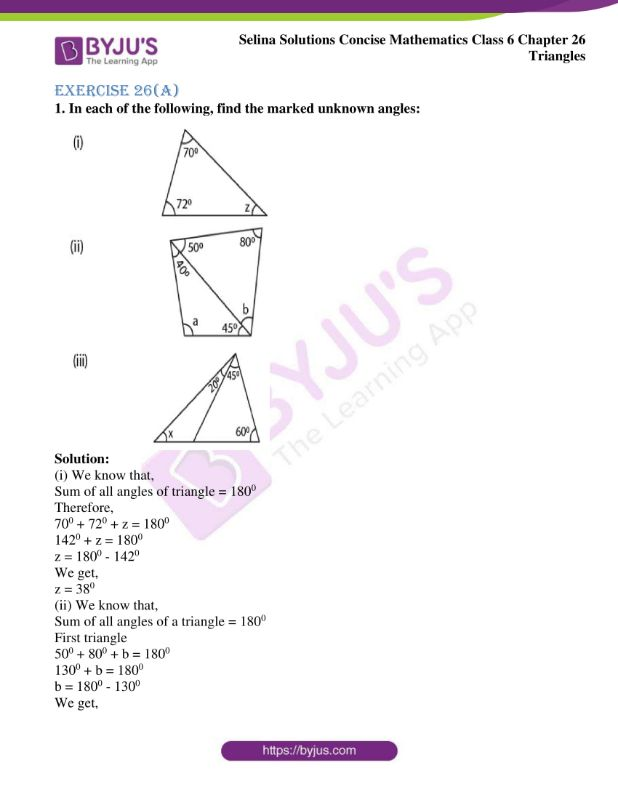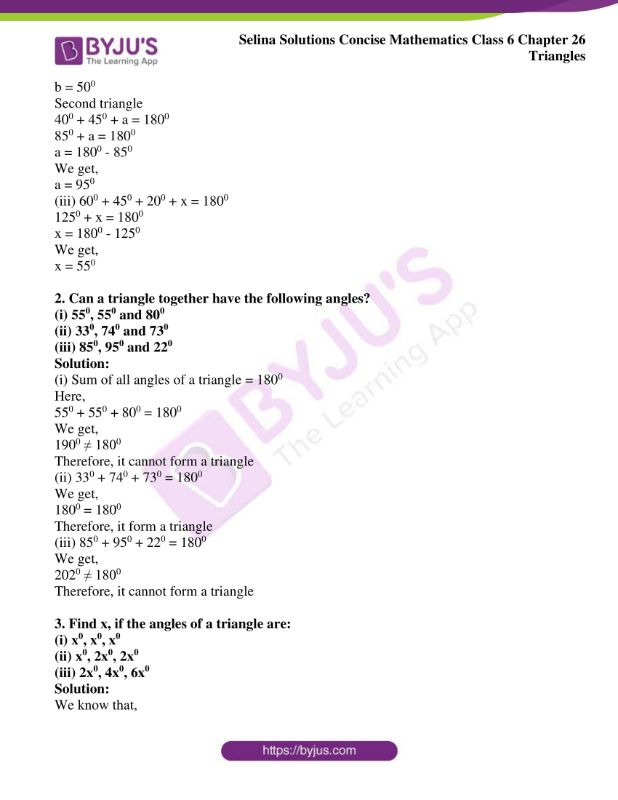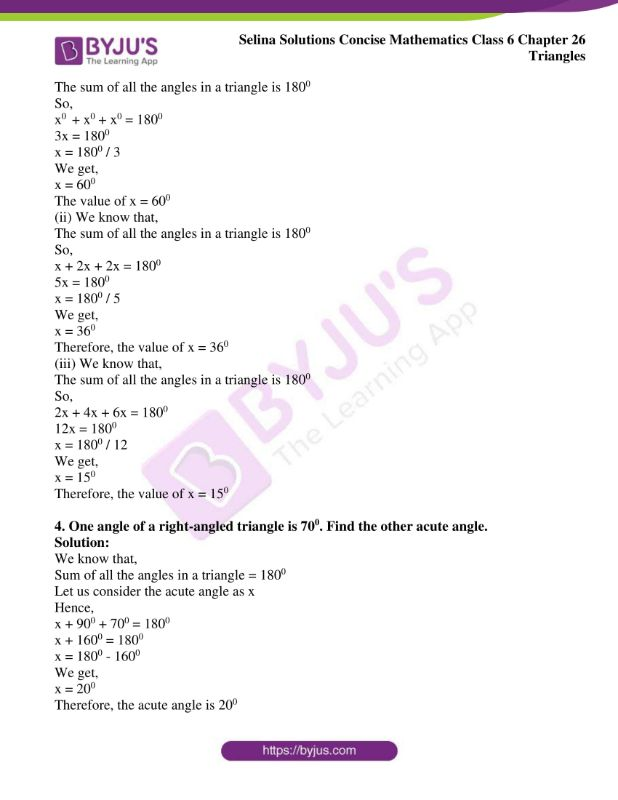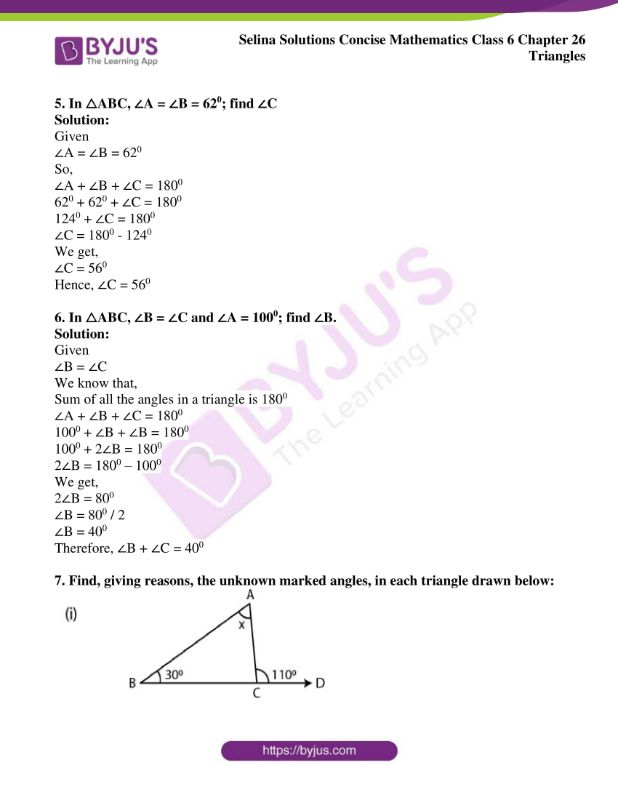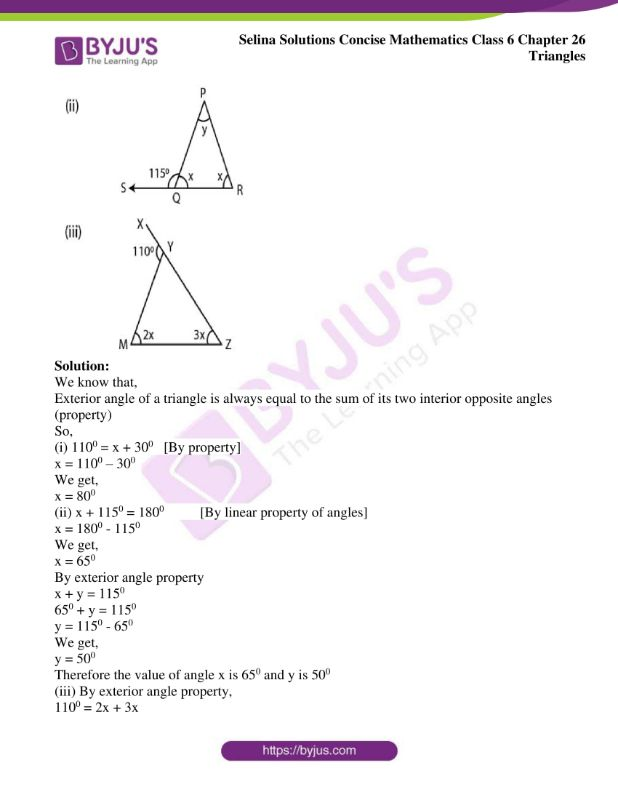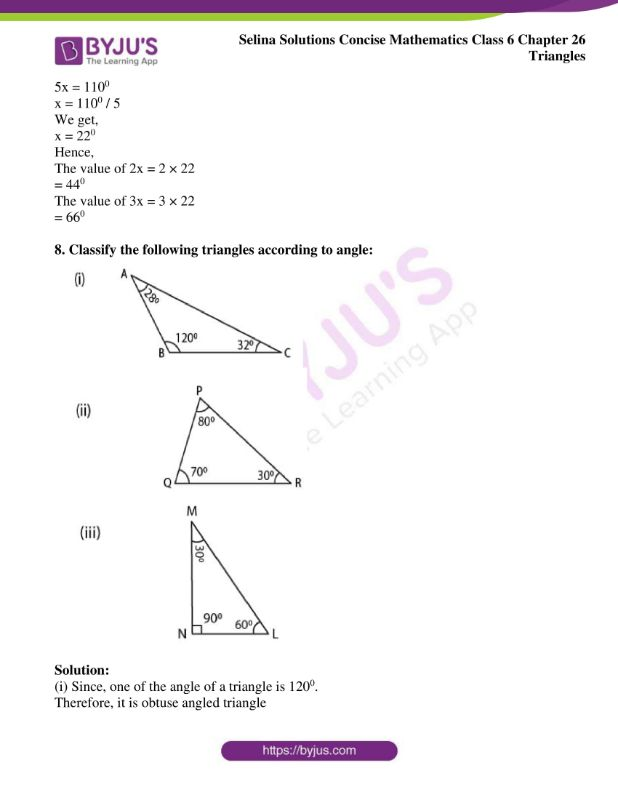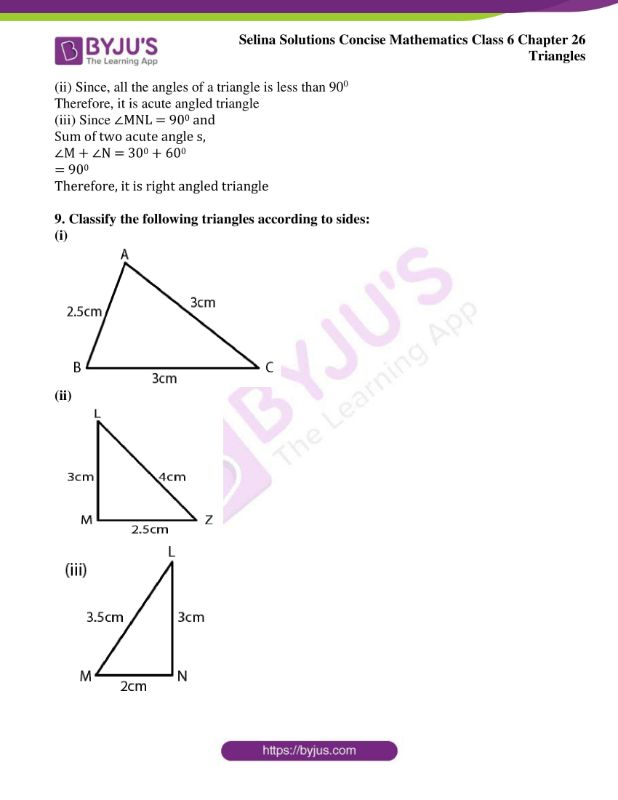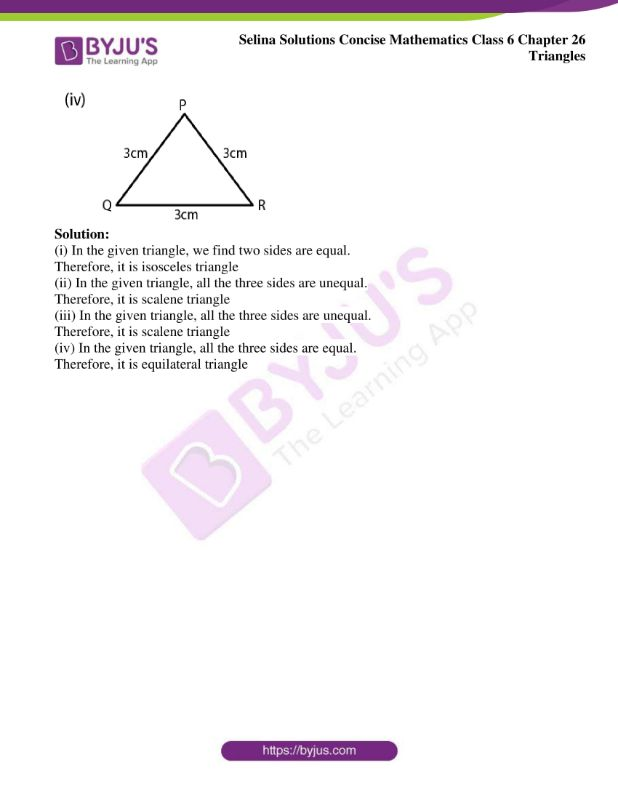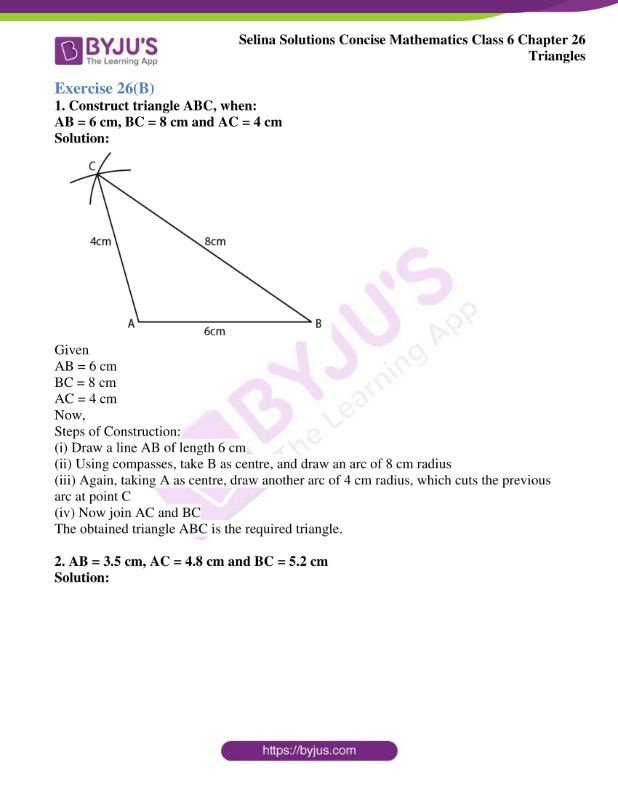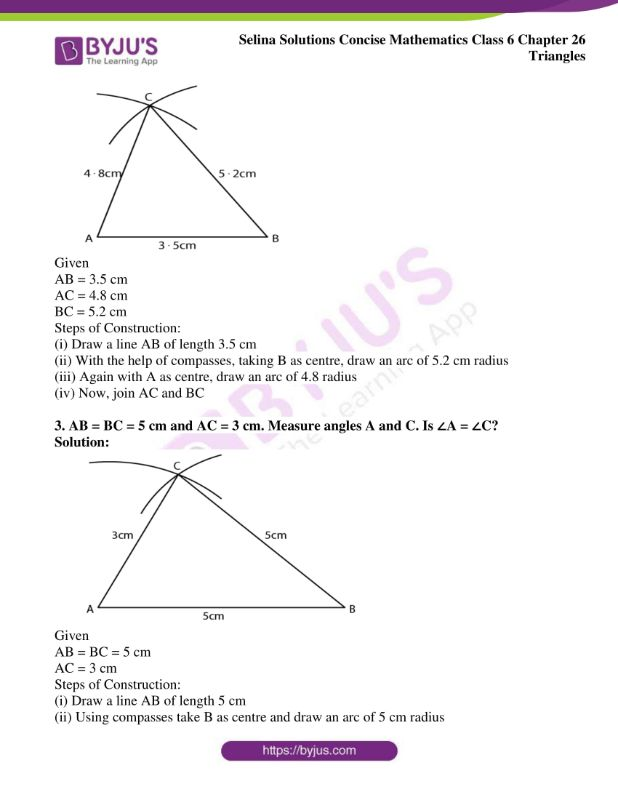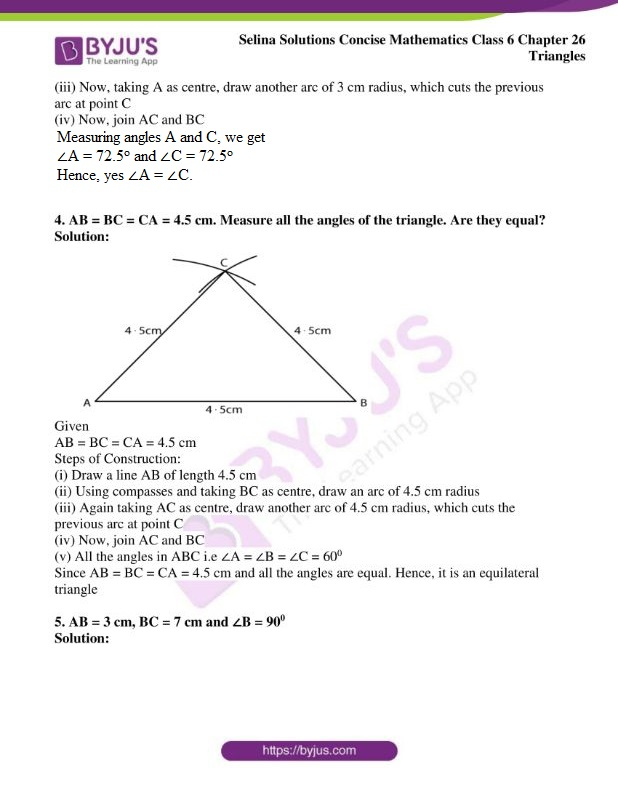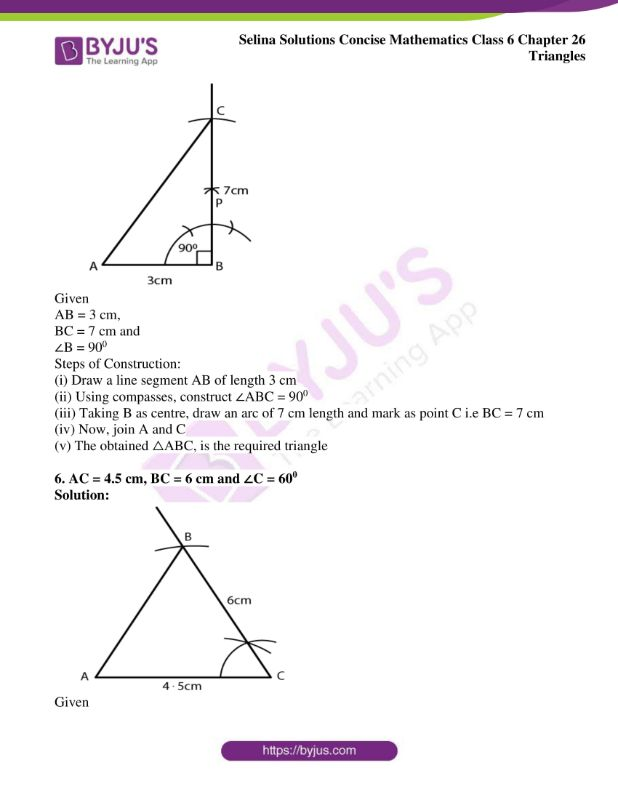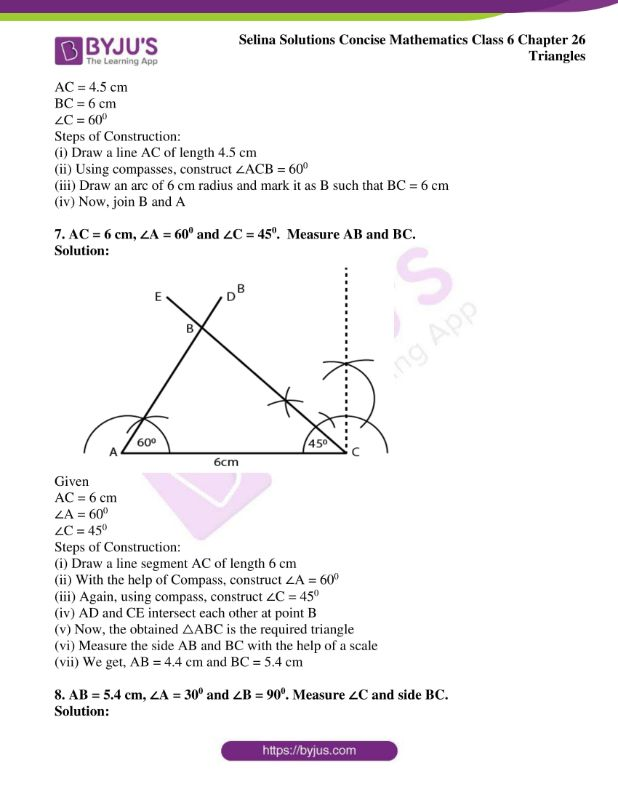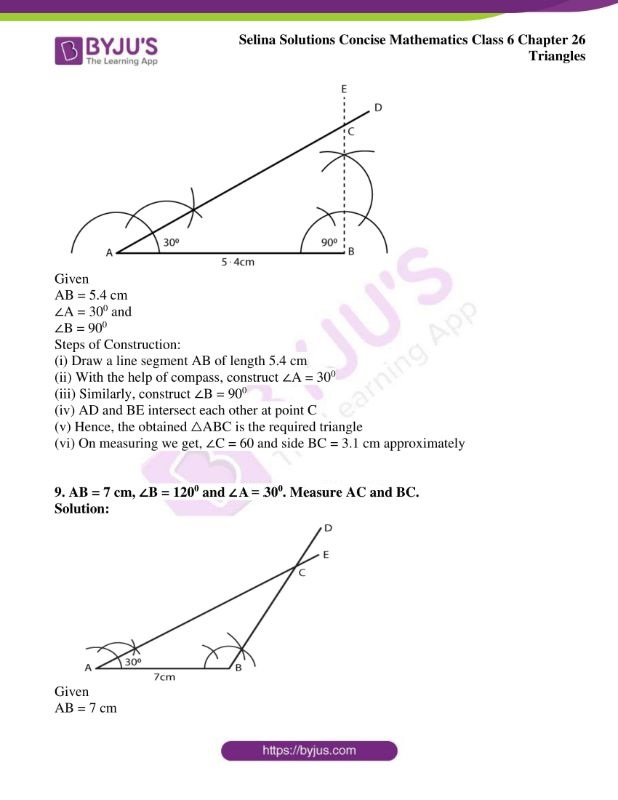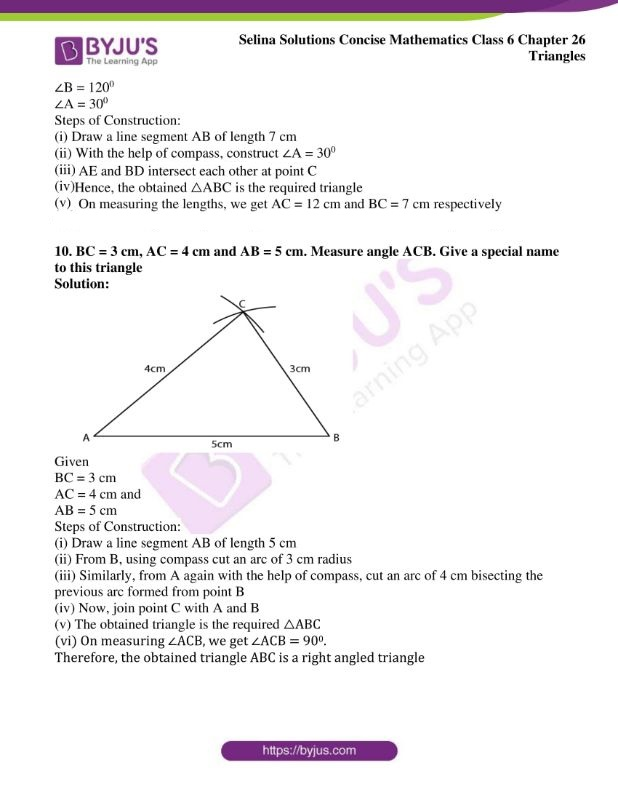### Exercises of Selina Solutions Concise Mathematics Class 6 Chapter 26: Triangles

Exercise 26(A) Solutions

Exercise 26(B) Solutions

## Access Selina Solutions Concise Mathematics Class 6 Chapter 26: Triangles

Exercise 26(A)

1. In each of the following, find the marked unknown angles: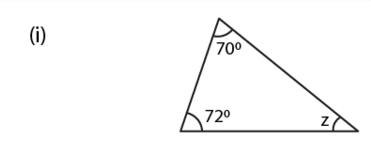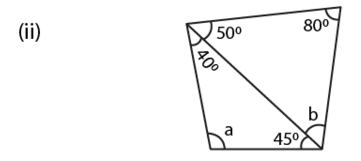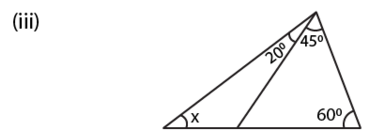Solution:

(i) We know that,

Sum of all angles of triangle = 1800

Therefore,

700 + 720 + z = 1800

1420 + z = 1800

z = 1800 – 1420

We get,

z = 380

(ii) We know that,

Sum of all angles of a triangle = 1800

First triangle

500 + 800 + b = 1800

1300 + b = 1800

b = 1800 – 1300

We get,

b = 500

Second triangle

400 + 450 + a = 1800

850 + a = 1800

a = 1800 – 850

We get,

a = 950

(iii) 600 + 450 + 200 + x = 1800

1250 + x = 1800

x = 1800 – 1250

We get,

x = 550

2. Can a triangle together have the following angles?

(i) 550, 550 and 800

(ii) 330, 740 and 730

(iii) 850, 950 and 220

Solution:

(i) Sum of all angles of a triangle = 1800

Here,

550 + 550 + 800 = 1800

We get,

1900 ≠ 1800

Therefore, it cannot form a triangle

(ii) 330 + 740 + 730 = 1800

We get,

1800 = 1800

Therefore, it form a triangle

(iii) 850 + 950 + 220 = 1800

We get,

2020 ≠ 1800

Therefore, it cannot form a triangle

3. Find x, if the angles of a triangle are:

(i) x0, x0, x0

(ii) x0, 2x0, 2x0

(iii) 2x0, 4x0, 6x0

Solution:

We know that,

The sum of all the angles in a triangle is 1800

So,

x0 + x0 + x0 = 1800

3x = 1800

x = 1800 / 3

We get,

x = 600

The value of x = 600

(ii) We know that,

The sum of all the angles in a triangle is 1800

So,

x + 2x + 2x = 1800

5x = 1800

x = 1800 / 5

We get,

x = 360

Therefore, the value of x = 360

(iii) We know that,

The sum of all the angles in a triangle is 1800

So,

2x + 4x + 6x = 1800

12x = 1800

x = 1800 / 12

We get,

x = 150

Therefore, the value of x = 150

4. One angle of a right-angled triangle is 700. Find the other acute angle.

Solution:

We know that,

Sum of all the angles in a triangle = 1800

Let us consider the acute angle as x

Hence,

x + 900 + 700 = 1800

x + 1600 = 1800

x = 1800 – 1600

We get,

x = 200

Therefore, the acute angle is 200

5. In △ABC, ∠A = ∠B = 620; find ∠C

Solution:

Given

∠A = ∠B = 620

So,

∠A + ∠B + ∠C = 1800

620 + 620 + ∠C = 1800

1240 + ∠C = 1800

∠C = 1800 – 1240

We get,

∠C = 560

Hence, ∠C = 560

6. In △ABC, ∠B = ∠C and ∠A = 1000; find ∠B.

Solution:

Given

∠B = ∠C

We know that,

Sum of all the angles in a triangle is 1800

∠A + ∠B + ∠C = 1800

1000 + ∠B + ∠B = 1800

1000 + 2∠B = 1800

2∠B = 1800 – 1000

We get,

2∠B = 800

∠B = 800 / 2

∠B = 400

Therefore, ∠B + ∠C = 400

7. Find, giving reasons, the unknown marked angles, in each triangle drawn below: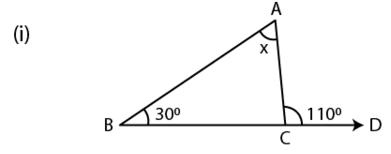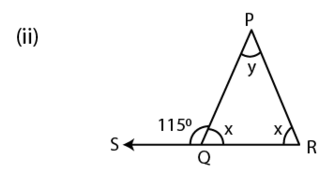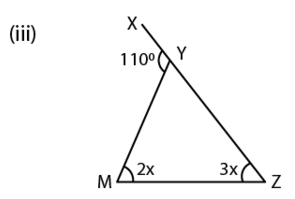Solution:

We know that,

Exterior angle of a triangle is always equal to the sum of its two interior opposite angles (property)

So,

(i) 1100 = x + 300 [By property]

x = 1100 – 300

We get,

x = 800

(ii) x + 1150 = 1800 [By linear property of angles]

x = 1800 – 1150

We get,

x = 650

By exterior angle property

x + y = 1150

650 + y = 1150

y = 1150 – 650

We get,

y = 500

Therefore the value of angle x is 650 and y is 500

(iii) By exterior angle property,

1100 = 2x + 3x

5x = 1100

x = 1100 / 5

We get,

x = 220

Hence,

The value of 2x = 2 × 22

= 440

The value of 3x = 3 × 22

= 660

8. Classify the following triangles according to angle: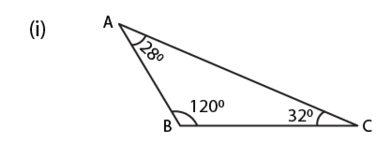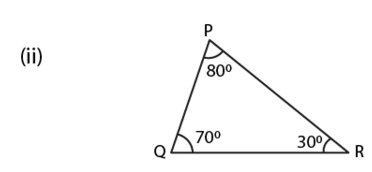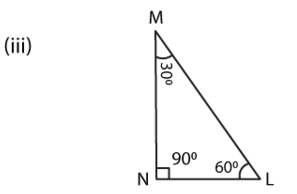Solution:

(i) Since, one of the angle of a triangle is 1200.

Therefore, it is obtuse angled triangle

(ii) Since, all the angles of a triangle is less than 900

Therefore, it is acute angled triangle

(iii) Since ∠MNL = 900 and

Sum of two acute angle s,

∠M + ∠N = 300 + 600

= 900

Therefore, it is right angled triangle

9. Classify the following triangles according to sides:

(i)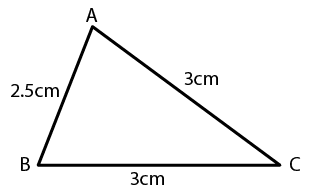(ii)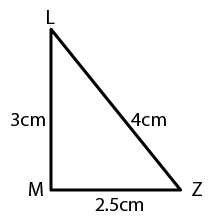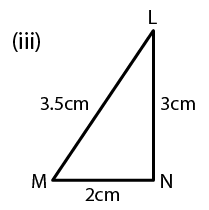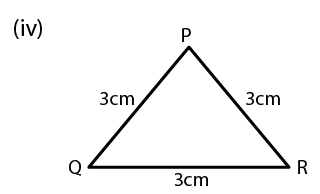Solution:

(i) In the given triangle, we find two sides are equal.

Therefore, it is isosceles triangle

(ii) In the given triangle, all the three sides are unequal.

Therefore, it is scalene triangle

(iii) In the given triangle, all the three sides are unequal.

Therefore, it is scalene triangle

(iv) In the given triangle, all the three sides are equal.

Therefore, it is equilateral triangle

Exercise 26(B)

1. Construct triangle ABC, when:

AB = 6 cm, BC = 8 cm and AC = 4 cm

Solution: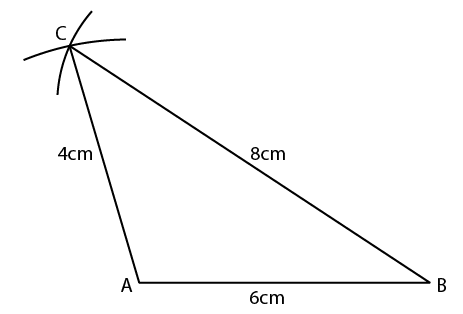Given

AB = 6 cm

BC = 8 cm

AC = 4 cm

Now,

Steps of Construction:

(i) Draw a line AB of length 6 cm

(ii) Using compasses, take B as centre, and draw an arc of 8 cm radius

(iii) Again, taking A as centre, draw another arc of 4 cm radius, which cuts the previous arc at point C

(iv) Now join AC and BC

The obtained triangle ABC is the required triangle.

2. AB = 3.5 cm, AC = 4.8 cm and BC = 5.2 cm

Solution: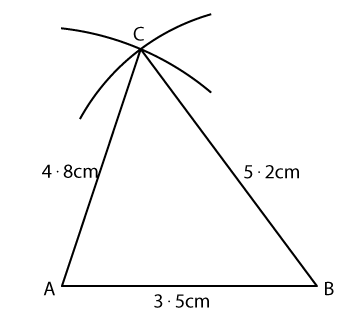Given

AB = 3.5 cm

AC = 4.8 cm

BC = 5.2 cm

Steps of Construction:

(i) Draw a line AB of length 3.5 cm

(ii) With the help of compasses, taking B as centre, draw an arc of 5.2 cm radius

(iii) Again with A as centre, draw an arc of 4.8 radius

(iv) Now, join AC and BC

3. AB = BC = 5 cm and AC = 3 cm. Measure angles A and C. Is ∠A = ∠C?

Solution: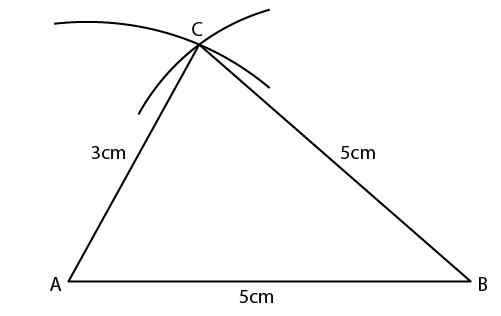Given

AB = BC = 5 cm

AC = 3 cm

Steps of Construction:

(i) Draw a line AB of length 5 cm

(ii) Using compasses take B as centre and draw an arc of 5 cm radius

(iii) Now, taking A as centre, draw another arc of 3 cm radius, which cuts the previous arc at point C

(iv) Now, join AC and BC

Measuring angles A and C, we get
∠A = 72.5o and ∠C = 72.5o
Hence, yes ∠A = ∠C.

4. AB = BC = CA = 4.5 cm. Measure all the angles of the triangle. Are they equal?

Solution: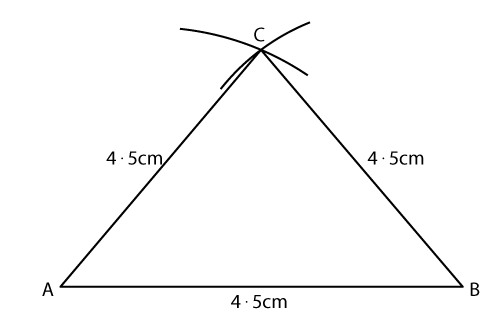Given

AB = BC = CA = 4.5 cm

Steps of Construction:

(i) Draw a line AB of length 4.5 cm

(ii) Using compasses and taking BC as centre, draw an arc of 4.5 cm radius

(iii) Again taking AC as centre, draw another arc of 4.5 cm radius, which cuts the previous arc at point C

(iv) Now, join AC and BC

(v) All the angles in ABC i.e ∠A = ∠B = ∠C = 600

Since AB = BC = CA = 4.5 cm and all the angles are equal. Hence, it is an equilateral triangle

5. AB = 3 cm, BC = 7 cm and ∠B = 900

Solution: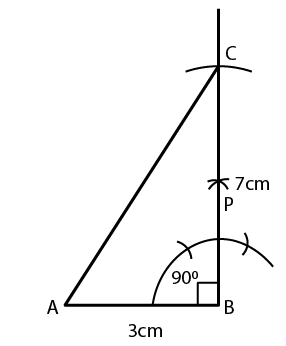Given

AB = 3 cm,

BC = 7 cm and

∠B = 900

Steps of Construction:

(i) Draw a line segment AB of length 3 cm

(ii) Using compasses, construct ∠ABC = 900

(iii) Taking B as centre, draw an arc of 7 cm length and mark as point C i.e BC = 7 cm

(iv) Now, join A and C

(v) The obtained △ABC, is the required triangle

6. AC = 4.5 cm, BC = 6 cm and ∠C = 600

Solution: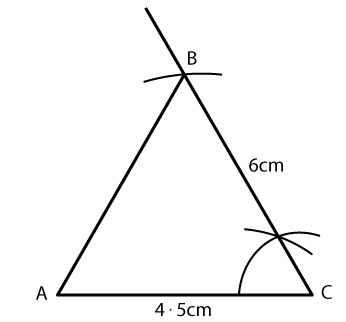Given

AC = 4.5 cm

BC = 6 cm

∠C = 600

Steps of Construction:

(i) Draw a line AC of length 4.5 cm

(ii) Using compasses, construct ∠ACB = 600

(iii) Draw an arc of 6 cm radius and mark it as B such that BC = 6 cm

(iv) Now, join B and A

7. AC = 6 cm, ∠A = 600 and ∠C = 450. Measure AB and BC.

Solution: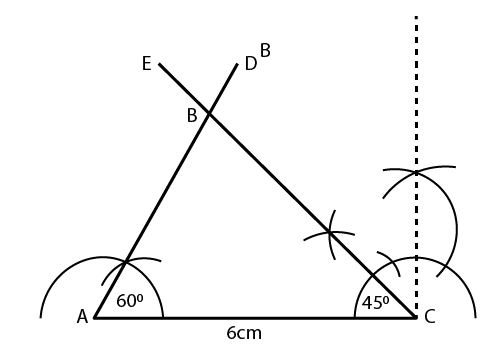Given

AC = 6 cm

∠A = 600

∠C = 450

Steps of Construction:

(i) Draw a line segment AC of length 6 cm

(ii) With the help of Compass, construct ∠A = 600

(iii) Again, using compass, construct ∠C = 450

(iv) AD and CE intersect each other at point B

(v) Now, the obtained △ABC is the required triangle

(vi) Measure the side AB and BC with the help of a scale

(vii) We get, AB = 4.4 cm and BC = 5.4 cm

8. AB = 5.4 cm, ∠A = 300 and ∠B = 900. Measure ∠C and side BC.

Solution: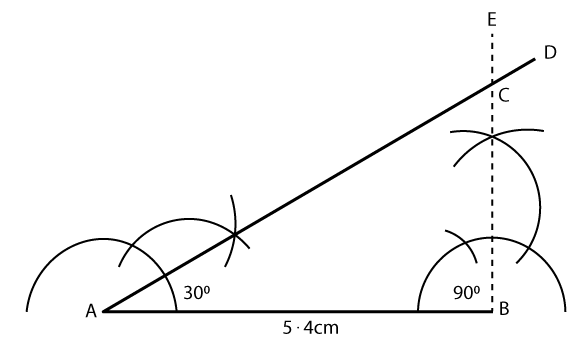Given

AB = 5.4 cm

∠A = 300 and

∠B = 900

Steps of Construction:

(i) Draw a line segment AB of length 5.4 cm

(ii) With the help of compass, construct ∠A = 300

(iii) Similarly, construct ∠B = 900

(iv) AD and BE intersect each other at point C

(v) Hence, the obtained △ABC is the required triangle

(vi) On measuring we get, ∠C = 60 and side BC = 3.1 cm approximately

9. AB = 7 cm, ∠B = 1200 and ∠A = 300. Measure AC and BC.

Solution: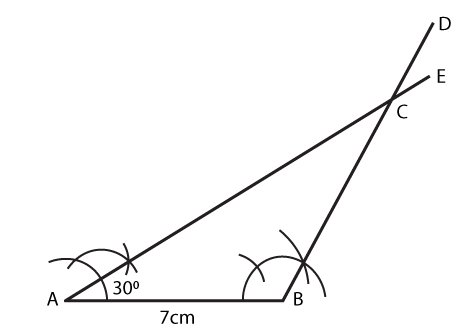Given

AB = 7 cm

∠B = 1200

∠A = 300

Steps of Construction:

(i) Draw a line segment AB of length 7 cm

(ii) With the help of a compass, construct ∠A = 300

(iii) AE and BD intersect each other at point C

(iv) Hence, the obtained △ABC is the required triangle

(v) On measuring the lengths, we get AC = 12 cm and BC = 7 cm respectively

10. BC = 3 cm, AC = 4 cm and AB = 5 cm. Measure angle ACB. Give a special name to this triangle

Solution: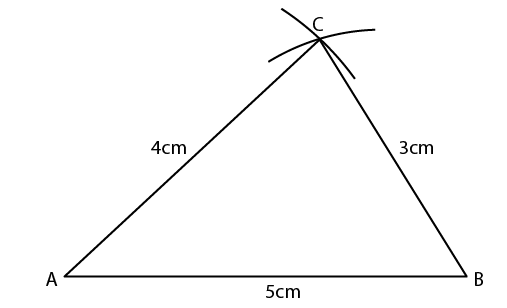Given

BC = 3 cm

AC = 4 cm and

AB = 5 cm

Steps of Construction:

(i) Draw a line segment AB of length 5 cm

(ii) From B, using compass cut an arc of 3 cm radius

(iii) Similarly, from A again with the help of compass, cut an arc of 4 cm bisecting the previous arc formed from point B

(iv) Now, join point C with A and B

(v) The obtained triangle is the required △ABC

(vi) On measuring ∠ACB, we get ∠ACB = 900.

Therefore, the obtained triangle ABC is a right angled triangle